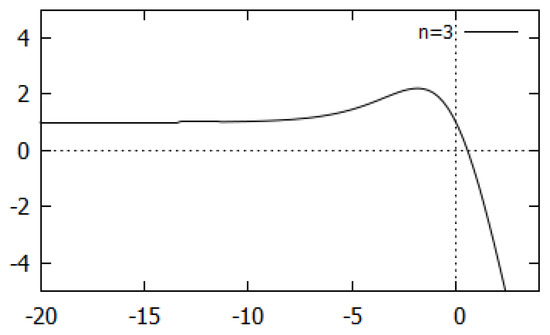Previous Article in Journal
Novel Spreadsheet Direct Method for Optimal Control Problems
Open AccessArticle

# Bifurcation and Chaos in Real Dynamics of a Two-Parameter Family Arising from Generating Function of Generalized Apostol-Type Polynomials

College of Engineering, Qassim University, Buraidah 52344, Saudi Arabia
Math. Comput. Appl. 2018, 23(1), 7; https://doi.org/10.3390/mca23010007
Received: 13 January 2018 / Revised: 30 January 2018 / Accepted: 1 February 2018 / Published: 3 February 2018
The aim of this paper is to investigate the bifurcation and chaotic behaviour in the two-parameter family of transcendental functions $f λ , n ( x ) = λ x ( e x + 1 ) n$ , $λ > 0$ , $x ∈ R$ , $n ∈ N \ { 1 }$ which arises from the generating function of the generalized Apostol-type polynomials. The existence of the real fixed points of $f λ , n ( x )$ and their stability are studied analytically and the periodic points of $f λ , n ( x )$ are computed numerically. The bifurcation diagrams and Lyapunov exponents are simulated; these demonstrate chaotic behaviour in the dynamical system of the function $f λ , n ( x )$ for certain ranges of parameter $λ$ . View Full-Text
Keywords:
Show FiguresFigure 1

MDPI and ACS Style

Sajid, M. Bifurcation and Chaos in Real Dynamics of a Two-Parameter Family Arising from Generating Function of Generalized Apostol-Type Polynomials. Math. Comput. Appl. 2018, 23, 7.

Show more citation formats Show less citations formats
Note that from the first issue of 2016, MDPI journals use article numbers instead of page numbers. See further details here.

1